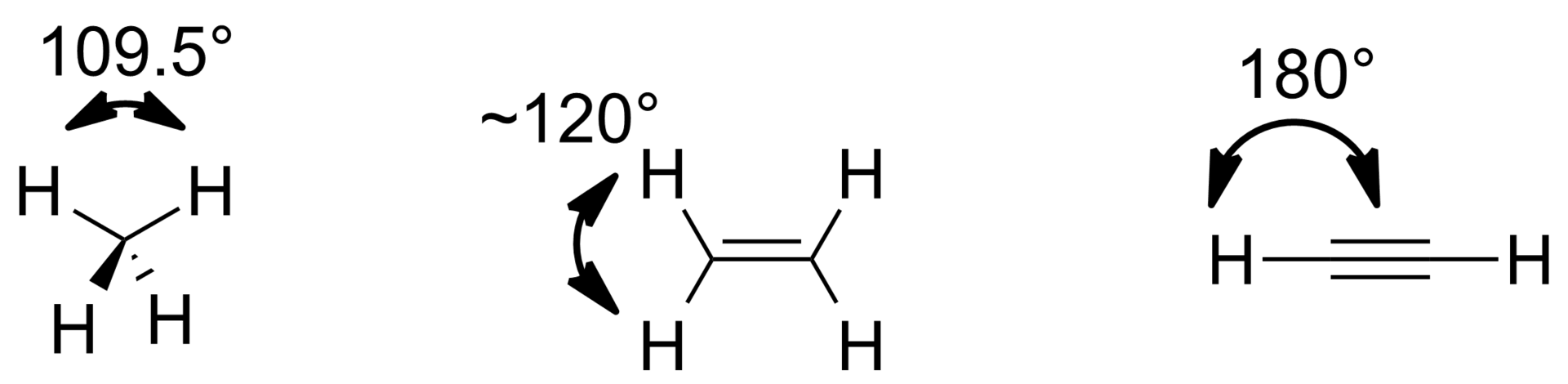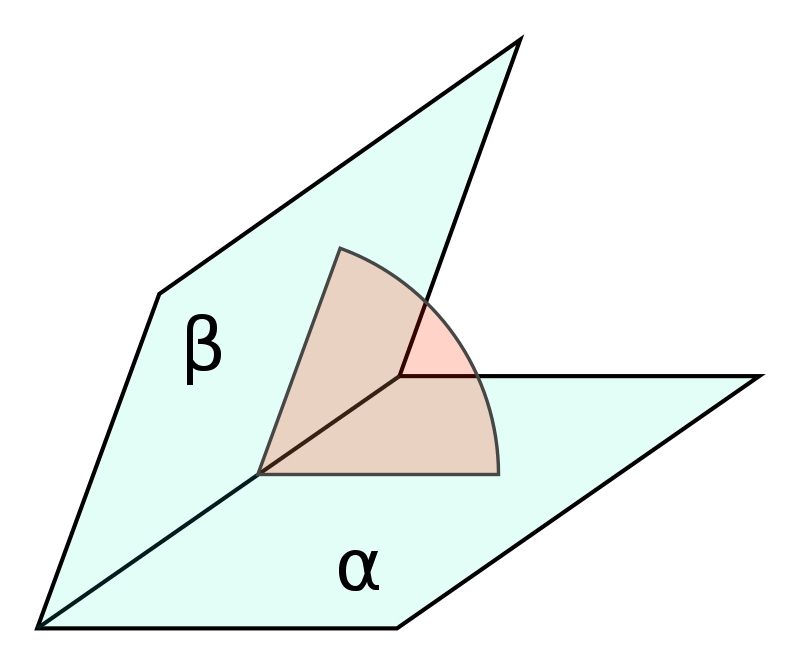# What is the Difference Between Bond Angle and Torsion Angle

The main difference between bond angle and torsion angle is that bond angle refers to the angle between two adjacent chemical bonds in a molecule, whereas torsion angle refers to the angle between two intersecting planes in a molecule.

Bond angle and torsion angle are both measures of the spatial arrangement of atoms in a molecule, but they refer to different aspects of molecular geometry.

### Key Areas Covered

1. What is a Bond Angle
– Definition, Features, Spatial Arrangement
2. What is a Torsion Angle
– Definition, Features, Spatial Arrangement
3. Similarities – Bond Angle and Torsion Angle
– Outline of Common Features
4. Difference Between Bond Angle and Torsion Angle
– Comparison of Key Differences

### Key Terms

Bond Angle, Torsion Angle## What is a Bond Angle

A bond angle is an angle formed between two adjacent chemical bonds in a molecule. It is the angle formed by three atoms, with the central atom being the vertex of the angle. Atoms form chemical bonds by sharing or transferring electrons in order to achieve stability. The arrangement of these bonds in space gives rise to molecular geometry. The bond angle is determined by the repulsion between electron pairs surrounding the central atom. This repulsion is governed by the VSEPR theory. According to VSEPR, electron pairs in the valence shell of an atom repel each other, and the molecule adopts a shape that minimizes this repulsion. Thus, the bond angle is a result of electron pair repulsion and the desire for a molecule to achieve maximum stability.Figure 1: Bond Angles of Arbitrary Alkanes, Alkenes, and Alkynes

The bond angle plays a major role in determining the polarity of a molecule. Polarity arises due to the uneven distribution of electron density within a molecule. When the bond angles are symmetrical, as in non-polar molecules like carbon dioxide, the molecule is non-polar. However, if the bond angles are not symmetrical as in polar molecules like water, the molecule exhibits polarity.

The magnitude of the bond angles also influences the strength of the intermolecular forces. Intermolecular forces are attractive forces that exist between molecules and play a significant role in determining the physical properties of substances. Moreover, the bond angles affect the reactivity of a molecule. The spatial arrangement of atoms and bonds influences how molecules interact with other substances.

## What is Torsion Angle

Torsion angle refers to the angle formed between two planes within a molecule, providing insights into the conformational flexibility and spatial arrangement of its constituent atoms. To understand torsion angles, the concept of the dihedral plane should be first understood. A dihedral plane is a plane formed by three consecutive atoms and the bond connecting them. In simple terms, it is like a flat surface passing through the atoms in a molecule. The torsion angle then is the angle between two adjacent dihedral planes. This angle is measured by selecting two atoms from each plane and the two bonds connecting them. Torsion angles play a main role in describing the spatial orientation of atoms within a molecule.Figure 2: Dihedral Angle

One common example to illustrate the significance of torsion angles is the rotation around a carbon-carbon single bond like alkanes. In simple hydrocarbons like ethane, the torsion angle between the two methyl groups attached to the central carbon atom can vary, leading to different confirmations. In the staggered conformation, the torsion angle is 180 degrees, resulting in maximum separation between the methyl groups. This conformation is energetically favored due to the reduced steric hindrance between atoms. On the other hand, in the eclipsed conformation, the torsion angle is 0 degrees, and the methyl groups are aligned directly opposite each other, resulting in increased steric hindrance and higher energy.

Torsion angles become more significant in complex biomolecules like proteins and DNA. Torsion angles also affect the conformational energy of a molecule. Different conformations have varying energy levels, and the torsion angles play a key role in determining these energy landscapes.

## Similarities Between Bond Angle and Torsion Angle

• Bond angles and torsion angles are geometric parameters that describe the spatial arrangement of atoms within a molecule.
• Both bond angles and torsion angles significantly impact the shape of a molecule.
• They are influenced by electron repulsion.
• Both influence various molecular properties.

## Difference Between Bond Angle and Torsion Angle

### Definition

The bond angle refers to the angle between two adjacent chemical bonds in a molecule, whereas the torsion angle refers to the angle between two intersecting planes in a molecule.

### Spatial Arrangement

Bond angles provide information about the orientation of adjacent bonds in a molecule and the overall molecular geometry. Torsion angles, on the other hand, provide information about the spatial arrangement of atoms within a molecule by describing the angle between two planes formed by consecutive atoms.

### Representation of Conformation

Bond angles provide a static representation of a molecule’s geometry, showing fixed angles between adjacent bonds, while torsion angles represent the flexibility and range of confirmations that a molecule can adapt by allowing rotations around specific bonds.

### Conclusion

The main difference between bond angle and torsion angle is that bond angle refers to the angle between two adjacent chemical bonds in a molecule,  whereas torsion angle refers to the angle between two intersecting planes in a molecule.

##### Reference:

1. Moss, G.P. “Torsion Angle.” Basic Terminology of Stereochemistry. De Gruyter
2. “Molecular geometry.” Wikipedia. Wikipedia Foundation.

##### Image Courtesy:

1. “Dihedral Angle” By CheCheOriginal: Luca Antonelli – This file was derived from Dihedral angle.png: (CC BY-SA 4.0) via Commons Wikimedia
2. “Angles of Alkane Alkene Alkyne” By B Levin13 – Own work (CC BY-SA 3.0) via Commons Wikimedia